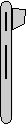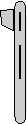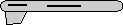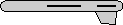# Maths - Rotations using quaternions - Samples in 90 degree steps

## Sample Rotations

In order to try to explain things and give some examples we can try I thought it might help to show the rotations for a finite subset of the rotation group. We will use the set of rotations of a cube onto itself, this is a permutation group which gives 24 possible rotations as explaned on this page.

Heading applied first giving 4 possible orientations:

 reference orientationq = 1 rotate by 90 degrees about y axisq = 0.7071 + j 0.7071 rotate by 180 degrees about y axisq = j rotate by 270 degrees about y axisq = 0.7071 - j 0.7071 (equivilant rotation to: -0.7071 + j 0.7071)

Then apply attitude +90 degrees for each of the above: (note: that if we went on to apply bank to these it would just rotate between these values, the straight up and streight down orientations are known as singularities because they can be fully defined without using the bank value) post multiply above by 0.7071 + k 0.7071 to give:q = 0.7071 + k 0.7071q = 0.5 + i 0.5 + j 0.5 + k 0.5q = i 0.7071 +j 0.7071q = 0.5 - i 0.5 - j 0.5 + k 0.5

Or instead apply attitude -90 degrees (also a singularity): post multiply top row by 0.7071 - k 0.7071 to give:q = 0.7071 - k 0.7071 (equivilant rotation to: -0.7071 + k 0.7071)q = 0.5 - i 0.5 + j 0.5 - k 0.5q = -i 0.7071 + j 0.7071 (equivilant rotation to: i 0.7071 - j 0.7071)q = 0.5 + i 0.5 - j 0.5 - k 0.5

Normally we dont go beond attitude + or - 90 degrees because thes are singularities, instead apply bank +90 degrees: post multiply top row by 0.7071 + i 0.7071 to give:q = 0.7071 + i 0.7071q = 0.5 + i 0.5 + j 0.5 - k 0.5q = j 0.7071 - k 0.7071q = 0.5 + i 0.5 - j 0.5 + k 0.5

Apply bank +180 degrees: post multiply top row by i to give:q = iq = i 0.7071 - k 0.7071q = kq = i 0.7071 + k 0.7071

Apply bank -90 degrees: post multiply top row by 0.7071 - i 0.7071 to give:q = 0.7071 - i 0.7071 (equivilant rotation to: -0.7071 + i 0.7071)q = 0.5 - i 0.5 + j 0.5 + k 0.5q = j 0.7071 + k 0.7071q = 0.5 - i 0.5 - j 0.5 - k 0.5

encoding of these rotations in matricies is shown here.
encoding of these rotations in axis-angle is shown here.
encoding of these rotations in euler angles is shown here.